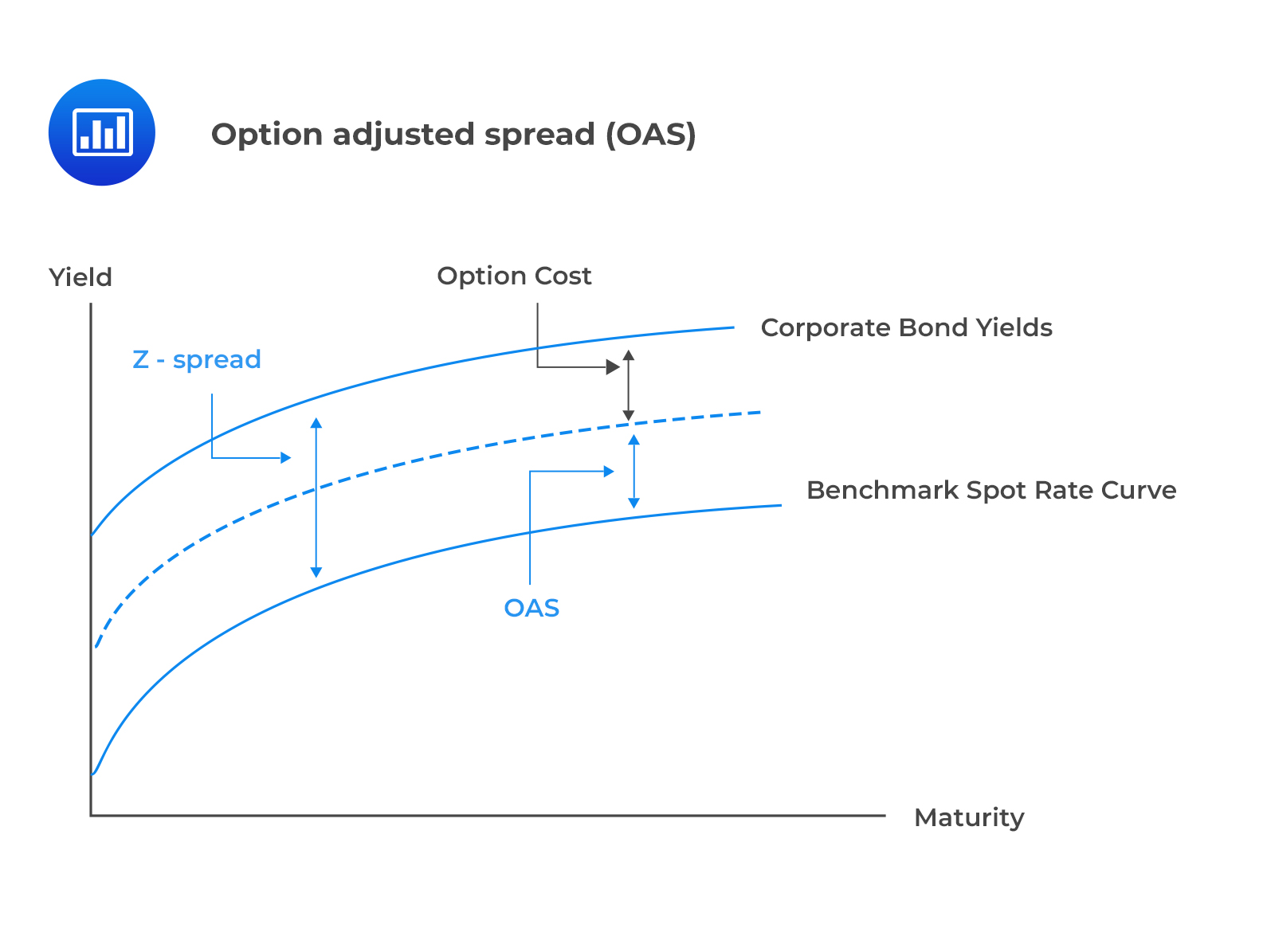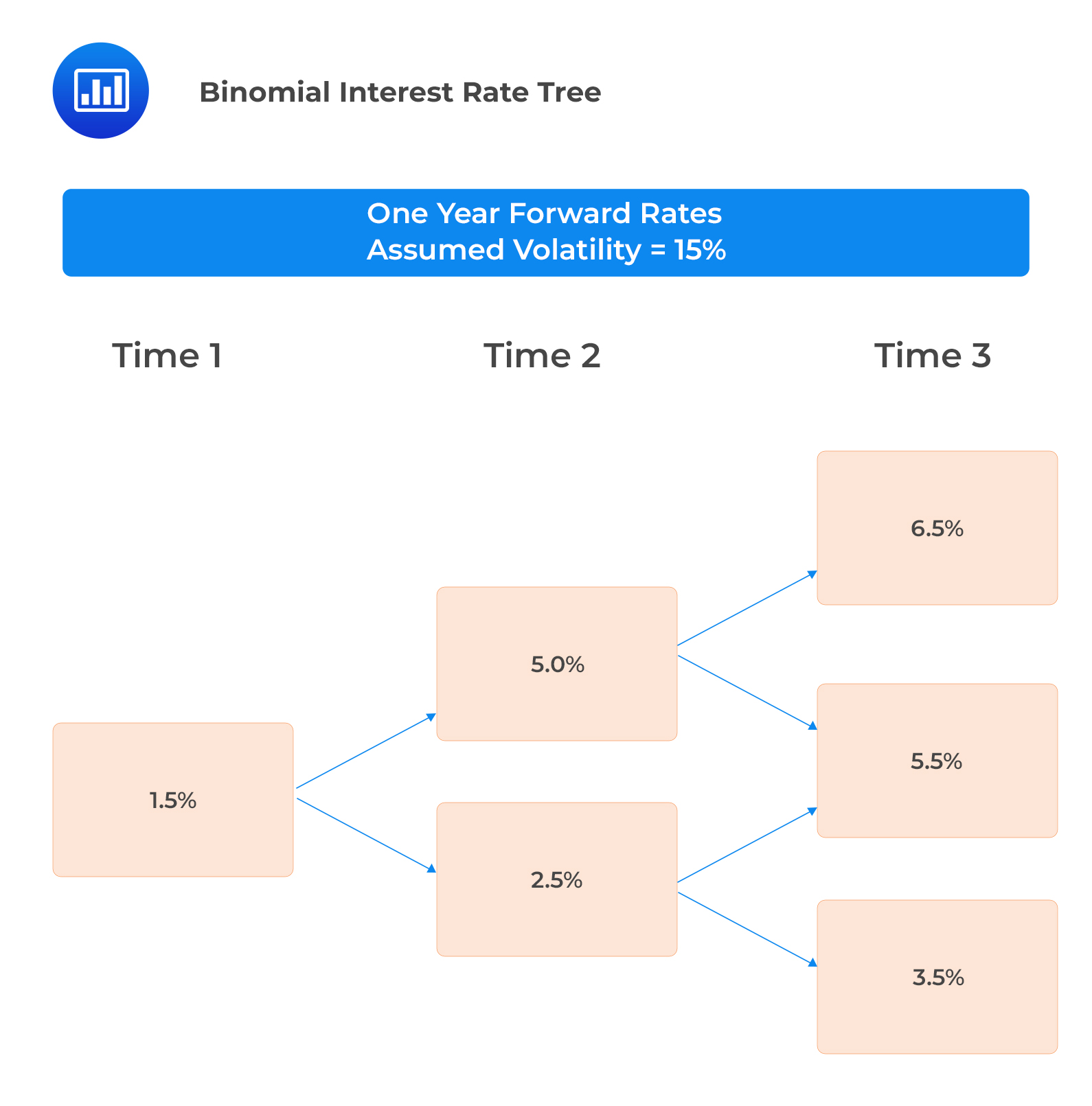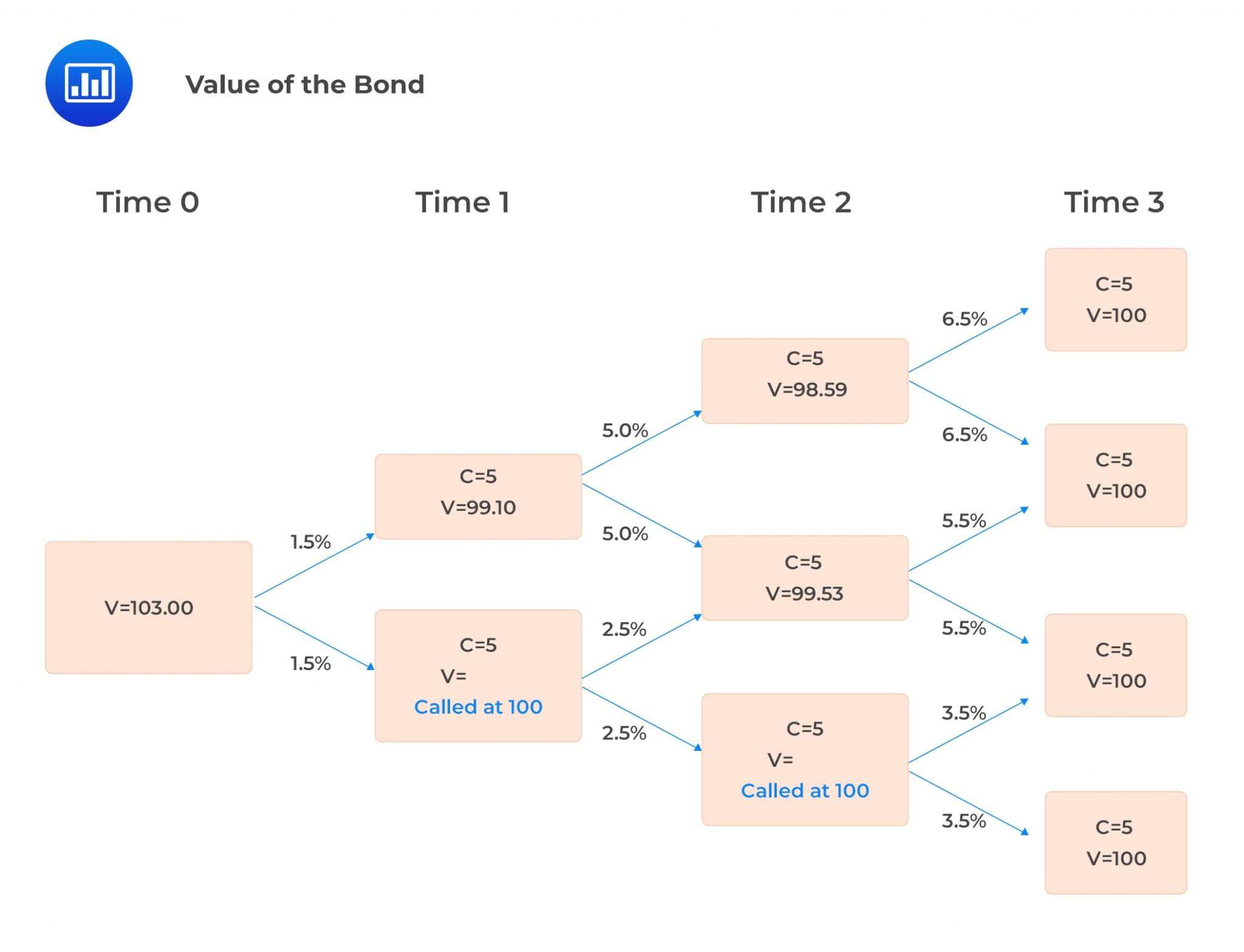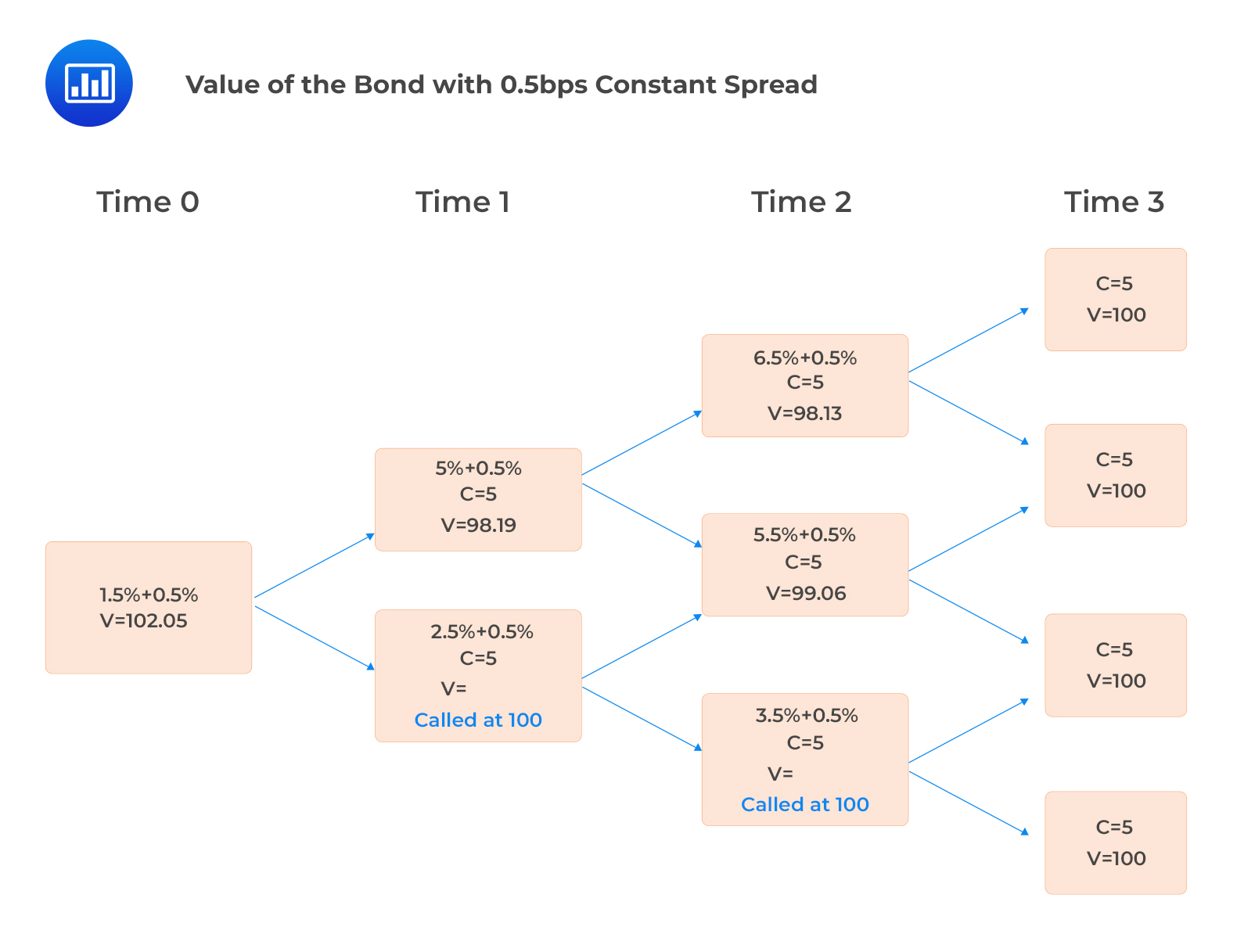A workable approach employed in constructing a suitable yield curve for risky bonds involves adding a fixed Z-spread to the one-year forward rates derived from the default-free spot yield curve.

Recall that Z-spread is the basis point spread that would need to be added to the default-free spot curve to equate the present value of cash flows to the market price of a risky bond.

The same approach can be used to value risky bonds with embedded options. Option-adjusted spread, which is the spread after adjusting for the options risk, is used in this case.

Option-adjusted spread (OAS) is the fixed spread added to the one-year forward rates on the interest rate tree that equates the arbitrage-free value and the market price of a risky bond with embedded options.

$$\text{Option adjusted spread (OAS)} = \text{Z-Spread} – \text{Option cost.}$$

The above relationship is illustrated in the following figure.OAS can be used to assess bond relative values. Two bonds with the same characteristics and credit quality must have the same OAS. Otherwise, the bond with the largest OAS is likely to be underpriced (cheap) relative to the bond with the smallest OAS.

Further, an OAS equal to that of a comparable bond indicates that the bond is fairly priced.

The following binomial interest rate tree has been calibrated, assuming an interest rate volatility of 15%.The OAS of a three-year 5% annual coupon risky bond, callable at par one year and two years from now priced at $102.05 can be computed as follows: The value of the bond using the above interest rate tree is$103.00, as shown in the following figure:We need to compute the OAS that justifies the current market price of $102.05 by trial and error. If we add a constant spread (OAS) of 50 bps to each one-period forward rate, we obtain a value of$102.05, which is equal to the current market price, as shown below:## Question

The following information relates to two risky callable bonds with similar characteristics and credit quality.

$$\begin{array}{c|c} \textbf{Bond} & \textbf{OAS} \\ \hline X & 30\text{bps} \\ \hline Y & 20\text{bps} \end{array}$$

Relative to bond Y, bond X is most likely:

1. Underpriced.
2. Fairly priced.
3. Overpriced.

#### Solution

An OAS higher than that of a comparable bond indicates that the bond is likely underpriced relative to the other.

In this case, bond X has the largest OAS, indicating that it is likely to be underpriced (cheap) relative to the bond Y with the smallest OAS.

Reading 30: Valuation and Analysis of Bonds with Embedded Options

Shop CFA® Exam Prep

Offered by AnalystPrepLevel I
Level II
Level III
All Three Levels
Featured Shop FRM® Exam PrepFRM Part I
FRM Part II
FRM Part I & Part II
Learn with Us

Subscribe to our newsletter and keep up with the latest and greatest tips for success
Shop Actuarial Exams PrepExam P (Probability)
Exam FM (Financial Mathematics)
Exams P & FMGMAT® Complete CourseDaniel Glyn
2021-03-24
I have finished my FRM1 thanks to AnalystPrep. And now using AnalystPrep for my FRM2 preparation. Professor Forjan is brilliant. He gives such good explanations and analogies. And more than anything makes learning fun. A big thank you to Analystprep and Professor Forjan. 5 stars all the way!michael walshe
2021-03-18
Professor James' videos are excellent for understanding the underlying theories behind financial engineering / financial analysis. The AnalystPrep videos were better than any of the others that I searched through on YouTube for providing a clear explanation of some concepts, such as Portfolio theory, CAPM, and Arbitrage Pricing theory. Watching these cleared up many of the unclarities I had in my head. Highly recommended.Nyka Smith
2021-02-18
Every concept is very well explained by Nilay Arun. kudos to you man!2021-02-13